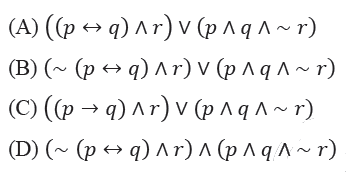# GATE | GATE-CS-2014-(Set-1) | Question 63

• Difficulty Level : Medium
• Last Updated : 11 Sep, 2017

Which one of the following propositional logic formulas is TRUE when exactly two of p, q, and r are TRUE?(A) A
(B) B
(C) C
(D) D

Explanation: Draw the truth table of three variables and output will be 1 (true) only when exactly two variables are 1 (true) else output will be 0 (false).
Therefore,

```p    q     r   Output (f)
0    0    0    0
0    0    1    0
0    1    0    0
0    1    1    1
1    0    0    0
1    0    1    1
1    1    0    1
1    1    1    0

```
```f = ( ~p ∧ q ∧ r) ∨ (p ∧ ~q ∧ r) ∨ (p ∧ q ∧ ~r)
f = {( ~p ∧ q ) ∨ (p ∧ ~q )} ∧ r  ∨ (p ∧ q ∧ ~r)
f = {(p ⊕ q )} ∧ r  ∨ (p ∧ q ∧ ~r)
f = {~ (p ↔ q )} ∧ r  ∨ (p ∧ q ∧ ~r)
```

Hence, option (b) is true.

Attention reader! Don’t stop learning now.  Practice GATE exam well before the actual exam with the subject-wise and overall quizzes available in GATE Test Series Course.

Learn all GATE CS concepts with Free Live Classes on our youtube channel.

Please comment below if you find anything wrong in the above post.

Quiz of this Question

My Personal Notes arrow_drop_up